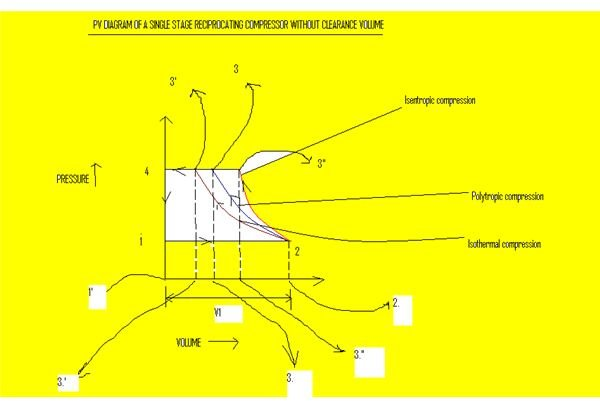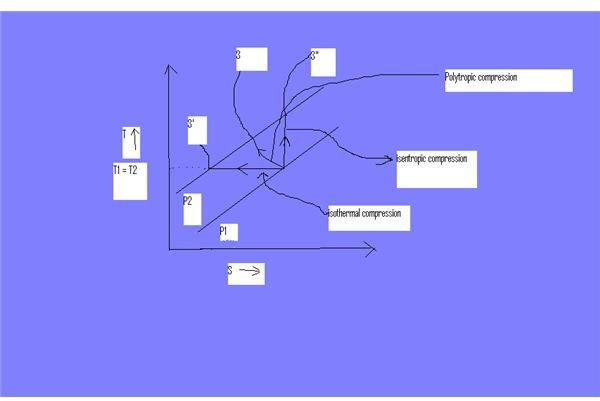Work Done By a Reciprocating Compressor

INTRODUCTION

The function of a compressor is to take a sufficient amount of fluid and to increase its pressure. Compressors may be classified into different categories. Some of them are the reciprocating compressor, rotary compressor, etc., Each have their own advantages and disadvantages. As we know, the reciprocating compressors may be used for high pressure applications.

Now let us consider the following for a single stage single acting reciprocating compressor without clearance volume.

Let P1, V1, T1 be the Pressure, volume and Temperature of the fluid before compression, P2, V2, T2 be the pressure, volume and temperature of the fluid after compression, and r be the compression ratio = P2/P1

Now let us discuss the PV and TS diagrams of a single acting, single stage reciprocating compressor without clearance volume,In this diagram, the path 2-3 represents the polytropic compression and the path 2-3′ represents the isothermal compression and the path 2-3" represents the isentropic compression.

From the above diagram it is clear that the air is drawn in by the cylinder during the suction stroke (1-2). The air is compressed during the compression stroke (2-3) at constant temperature (T1=T2). Now, the pressure rises from P1 to P2 from 2-3. The air will be delivered when sufficient pressure has been reached so that the valves can be opened. The delivery of air takes place from 2-3.

In brief:

1-2 : suction stroke (air is drawn into the cylinder)

2-3 : compression stroke (air gets compressed inside the cylinder)

3-4 : air is delivered under pressure

4-1 : delivery closes and the suction valve opens for admitting fresh air inside the cylinder

The compression may be isothermal, isentropic, or polytropic compression. Now let us discuss each in detail in the following paragraphs.

ISOTHERMAL COMPRESSION

Referring to the PV diagram, the isothermal compression follows the path 1-2-3′-4-1. It is clear that the volume of air delivered in this process is:

V2 = 3′-4.

The work done by the compressor per cycle can be given by the area under the curve 1-2-3′-4-1.

(ie) Work done W = Area 1-2-3′-4-1

The above area can be further split as follows:

W= Area 1′-4-3′-3.’ + Area 2-3′-3.’-2. – Area 1-1′-2-2.

= P2V2 + 2.3 P2V2 log (V1/V2) – P1V1 —————————————(1)

We know that for an isothermal process PV = constant

Therefore, P1V1 = P2V2

The equation (1) can be written as:

W= 2.3 P2V2 log (V1/V2)

= 2.3 P2V2 log (P2/P1)

Since P1V1 = P2V2 , Therefore V1/V2 = P2/P1

= 2.3 P2V2 log (r) ( where r – compression ratio = P2/P1)

Therefore the work done by the compressor is :

W = 2.3 m R T1 log(r) ( where m is mass of air delivered by the compressor per cycle)

(since P2V2 = P1V1 = m R T1)

POLYTROPIC COMPRESSION

Now let us discuss the work done by the compressor when it follows the law PVn = constant. The polytropic compression follows the path 1-2-3-4-1.

The volume of air delivered is V2= 3-4

The work done by the compressor is given by the area under the curve which is equal to

W= Area 1-2-3-4-1

= Area 3-4-1′-3. + Area 2-3-3.-2. – Area 2-1-1′-2.

= P2V2 + (P2V2 – P1V1)/(n-1) – P1V1

= (P2V2(n-1) + P2V2 – P1V1 – P1V1(n-1))/(n-1)

= (nP2V2 – P2V2 + P2V2 – P1V1 – nP1V1 + P1V1)/(n-1)

= (n/n-1)(P2V2-P1V1) —————————————–(2)

= (n/n-1)P1V1(P2V2/P1V1 – 1) ————————————–(3)

We know that

for a polytroic compression PVn = constant

P1V1n = P2V2n

or V2/V1 = (P1/P2)1/n

Therefore the equation (3) can be re-written as:

W = (n/n-1)P1V1((P2/P1)1/n – 1 )

W = (n/n-1)P1V1((P2/P1)(n-1)/n

is the work done bye the compressor.

(or) The equation (2) can be re-written as:

W= (n/n-1)P2V2(1 – P1V1/P2V2)

= (n/n-1)P2V2(1 – (P1/P2)n-1/n )

since

P1V1n = P2V2n

or V2/V1 = (P1/P2)1/n or V1/V2 = (P2/P1)1/n

Therefore W = (n/n-1) m R T2 (1 – T1/T2)

The work done by the compressor is

W = (n/n-1) m R ( T2-T1)

since, for a polytropic process PVn = constant

consider PV = RT Implies V = RT/P

Therefore P ( RT/P)n = constant

or T/(P)n-1/n =constant.

Therefore T1/T2 = (P1/P2)n-1/n

ISENTROPIC COMPRESSION

The compression follows the path 1-2-3"-4-1. The volume of air delivered being 3"-4.

V2= 3"-4

This is very similar to polytropic compression except the polytropic index n is replaced by γ. Therefore the work done by the compressor is

W = γ/(γ-1) m R (T2- T1) ———————————(4)

We know that

R = cp – cv and γ = cp / cv (where R- gas constant, cp -specific heat capacity at constant pressure, cv – specific heat capacity at constant volume respectively)

R can be re-written as

R = cp (1-1/γ) = cp ((γ-1)/γ)

Therefore equation (4) can be re-written as:

W= γ/(γ-1) m cp ((γ-1)/γ) (T2 – T1)

The work done by the compressor is

W = m cp (T2 – T1)

which means that the work done is equal to the heat required for raising the temperature from T1 to T2 at a constant pressure.

POWER REQUIRED

The power required to drive a single acting reciprocating compressor is

P = W Nw / 60

where,

N – the speed of the compressor in rpm,

Nw – the no. of working strokes per minute

Nw = N for a single acting compressor,

= 2N for a double acting compressor.

and W denotes the work done by the compressor.

In general,

Isothermal power = W (isothermal compression ) Nw / 60 watts

Polytropic power = W (polytropic compression) Nw / 60 watts

Isentropic power = W ( Isentropic compression) Nw / 60 watts